# 详解逆卷积操作–Up-sampling with Transposed Convolution

• 2
• 969 views
• A+

## 方法介绍

• 卷积是有一种，多对一的关系；
• 逆卷积是有一种，一对多的关系；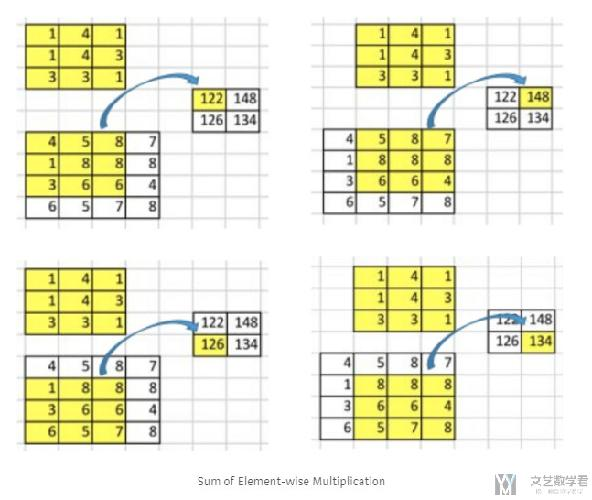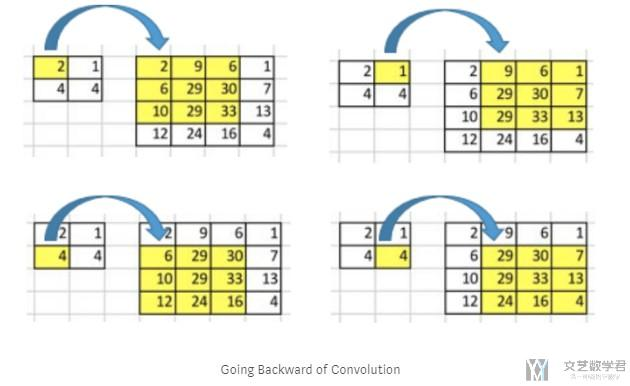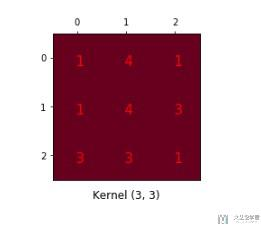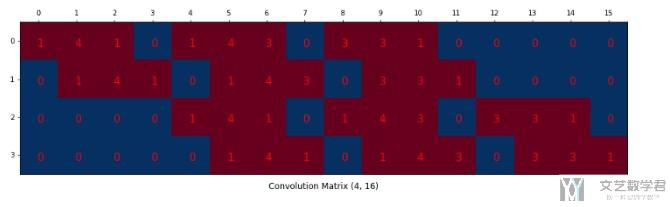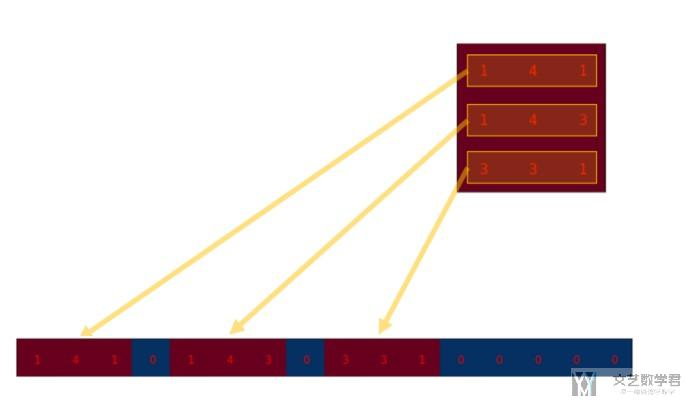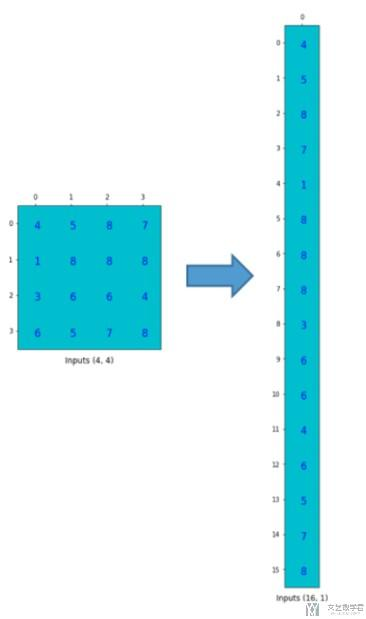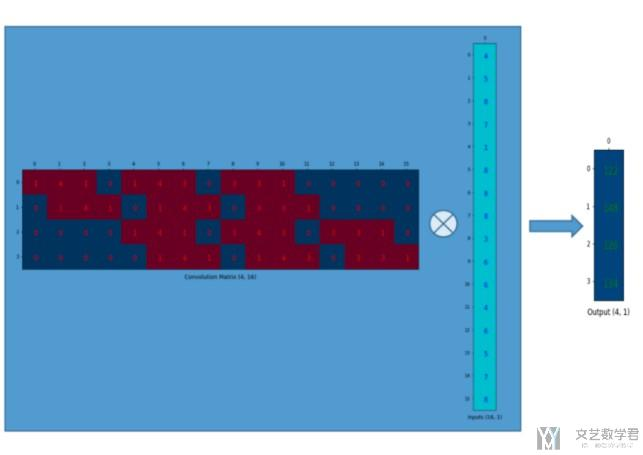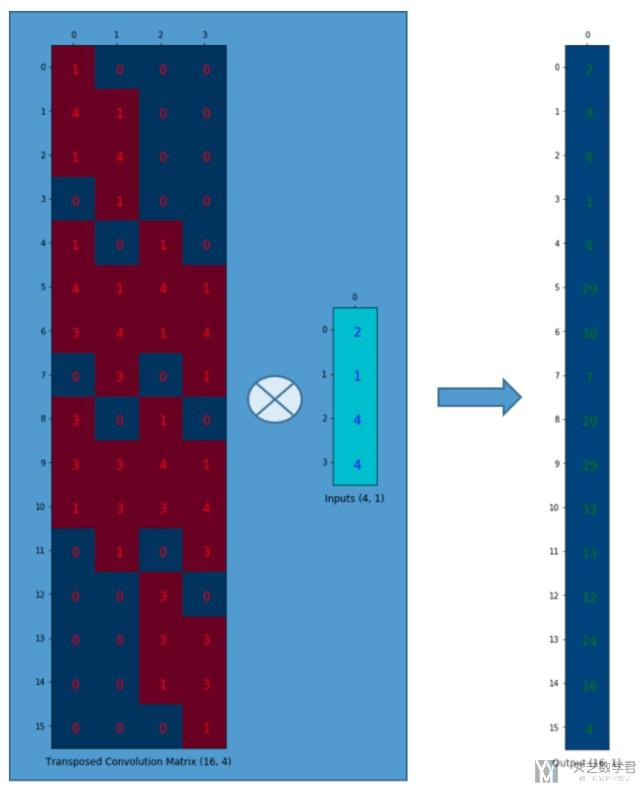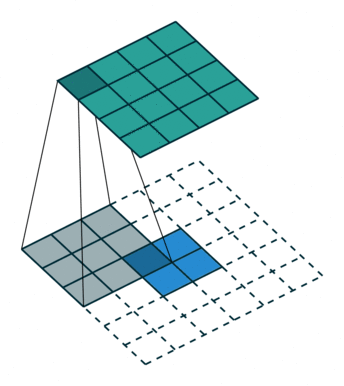## 实现结果

### 加载数据集

1. import torch
2. import torch.nn as nn
3. import torchvision
4. import torchvision.transforms as transforms
5. # Device configuration
6. device = torch.device('cuda' if torch.cuda.is_available() else 'cpu')
7. # Hyper-parameters
8. num_epochs = 5
9. batch_size = 100
10. learning_rate = 0.001
11. # MNIST dataset
12. train_dataset = torchvision.datasets.MNIST(root='./',
13.                                            train=True,
14.                                            transform=transforms.ToTensor(),
16. test_dataset = torchvision.datasets.MNIST(root='./',
17.                                           train=False,
18.                                           transform=transforms.ToTensor())
21.                                            batch_size=batch_size,
22.                                            shuffle=True)
24.                                           batch_size=batch_size,
25.                                           shuffle=False)

### 定义网络结构

• Conv->MaxPool->MaxUnpool->UnConv
• 每一层图像大小的变化 28*28(input)->26*26(conv)->13*13(pool)->26*26(unpool)->28*28(output)
1. # 搭建网络
2. class CNNMNIST(nn.Module):
3.     def __init__(self):
4.         super(CNNMNIST,self).__init__()
8.         self.unconv1 = nn.ConvTranspose2d(in_channels=1, out_channels=1, kernel_size=3, stride=1, padding=0)
9.     def forward(self,x):
10.         # encode
11.         out1 = self.conv1(x)
12.         out = out1.clone()
13.         out,indices = self.pool1(out)
14.         # deocde
15.         out = self.unpool1(out,indices,output_size=out1.size())
16.         out = self.unconv1(out)
17.         return out
18. # 网络的初始化
19. model = CNNMNIST().to(device)
20. print(model)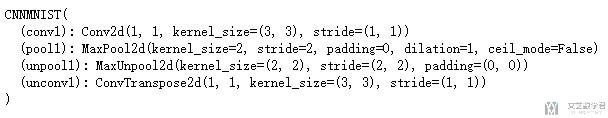### 网络的训练

1. # 定义优化器和损失函数
2. criterion = nn.MSELoss(reduction='mean')
4. # 进行训练
5. model.train()
7. for epoch in range(num_epochs):
8.     for i, (images, labels) in enumerate(train_loader):
9.         # Move tensors to the configured device
10.         images = images.to(device)
11.         # Forward pass
12.         outputs = model(images)
13.         loss = criterion(outputs, images)
14.         # Backward and optimize
16.         loss.backward()
17.         optimizer.step()
18.         if (i+1) % 100 == 0:
19.             # 计算Loss
20.             print ('Epoch [{}/{}], Step [{}/{}], Loss: {:.4f}'
21.                    .format(epoch+1, num_epochs, i+1, total_step, loss.item()))

### 重构的效果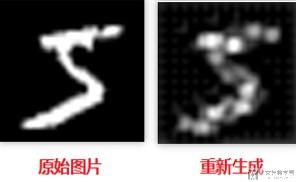### 看卷积和逆卷积的系数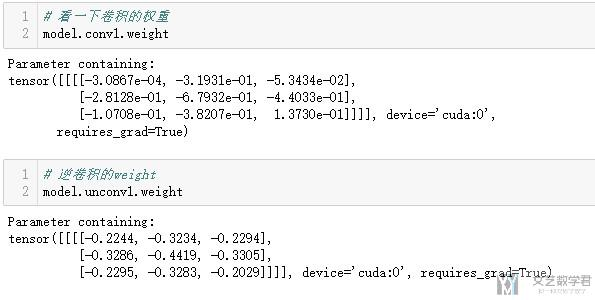• 微信公众号
• 关注微信公众号
•• QQ群
• 我们的QQ群号
•## 目前评论：2   其中：访客  1   博主  1

•clatterrr

正被卷积玩得死去活来….
话说卷积是组合数学的内容吧？还是具体数学…

•王 茂南 Admin

这个分类我也不是很懂, 我之前看卷积是与图像处理有关. 我这里写的是介绍关于深度学习中(CNN)中卷积的操作.